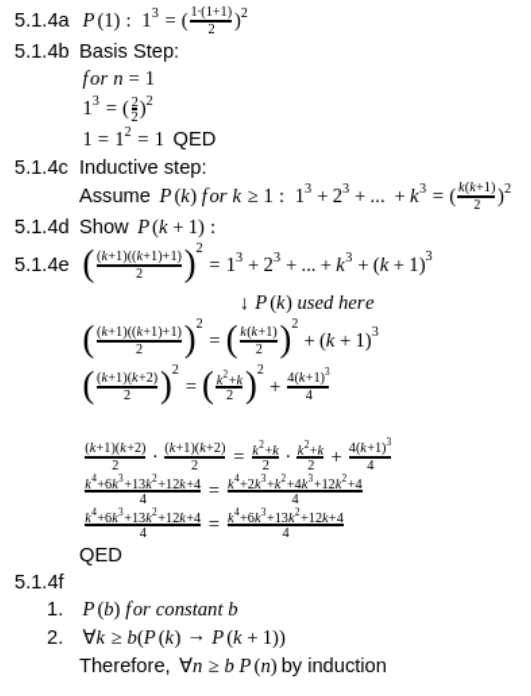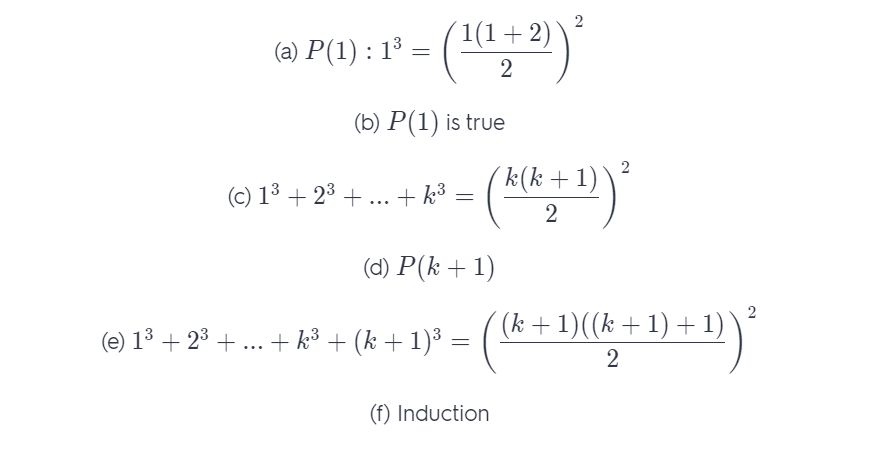# Let P(N) Be The Statement That 1^3 + 2^3

We thoroughly check each answer to a question to provide you with the most correct answers. Found a mistake? Let us know about it through the REPORT button at the bottom of the page.

Let P (n) be the statement that 1³ + 2³ + · · · + n³ = (n(n + 1)/2)² for the positive integer n.

a) What is the statement P (1)?

b) Show that P (1) is true, completing the basis step of the proof.

c) What is the inductive hypothesis?

d) What do you need to prove in the inductive step?

e) Complete the inductive step, identifying where you use the inductive hypothesis.

f ) Explain why these steps show that this formula is true whenever n is a positive integer.